#Function Repository Resource:

# DifferencesBy

Apply a function to neighboring pairs in a list

Contributed by: Bob Sandheinrich
 ResourceFunction["DifferencesBy"][list,f] applies f to successive neighboring pairs of element in list. ResourceFunction["DifferencesBy"][f] represents an operator form of ResourceFunction["DifferencesBy"].

## Details and Options

The result of ResourceFunction["DifferencesBy"][{e1,e2,e3,},f] is {f[e2,e1],f[e3,e2],}.
The output of ResourceFunction["DifferencesBy"] contains one fewer element than list.

## Examples

### Basic Examples

Find differences between images:

 In:=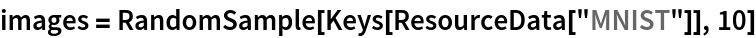Out=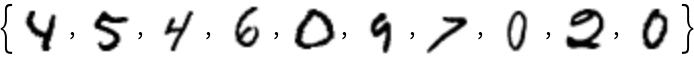In:=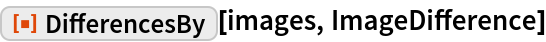Out=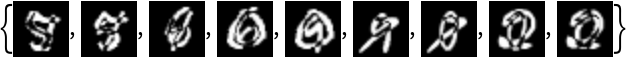Find the elements of each sublist that are absent from the proceeding sublist:

 In:=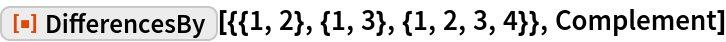Out=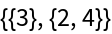### Scope

Create an operator:

 In:=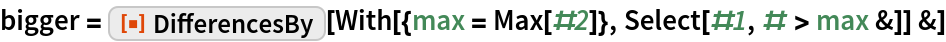Out=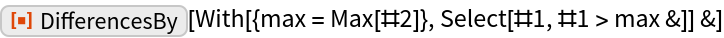In:=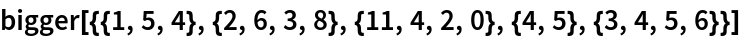Out=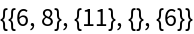### Applications

Find vocabulary differences:

 In:=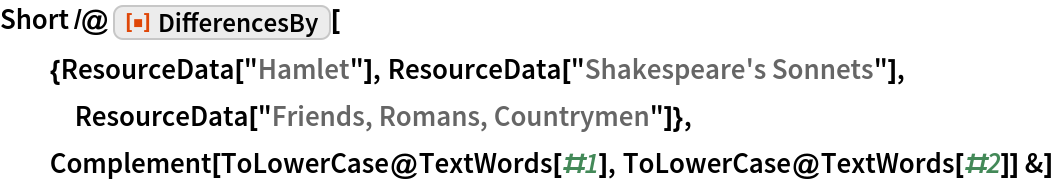Out=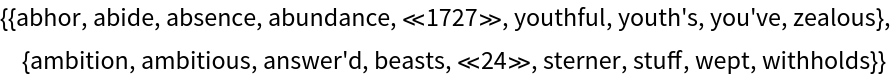Look at the growth of the string-based SubstitutionSystem:

 In:=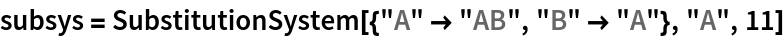Out=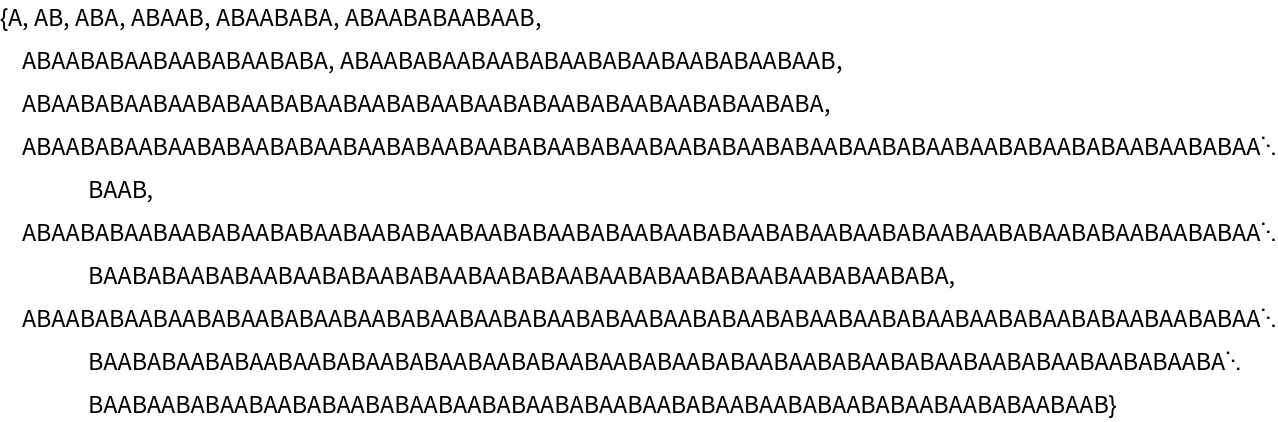In:=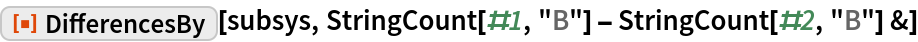Out=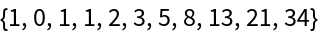### Properties and Relations

DifferencesBy is a generalization of Differences:

 In:=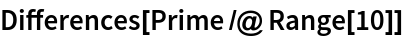Out=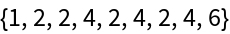Using Subtract as f gives the same result:

 In:=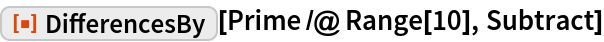Out=The function passed to DifferencesBy does not need to be subtraction:

 In:=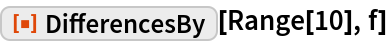Out=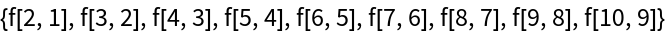In:=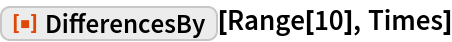Out=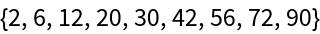## Author Notes

This could be extended to allow the Part-like arguments in Differences.